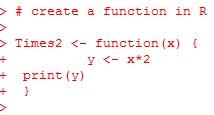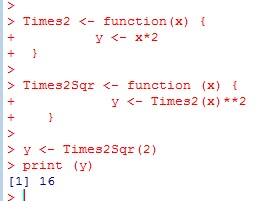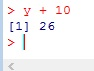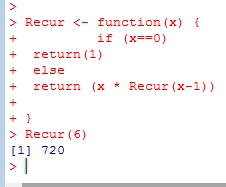# R: Create Functions in R

Learning to use functions in R will improve your programming skills greatly. The way I think of functions is I think of them as mini programs you create inside your program.

Below I create function called Times2. The syntax for creating a function is:

```FunctionName <- function(parameters) {
Action (body of the function)
}```You call the function simply by calling it’s name and giving it a parameter.You can even pass the function a vector## Nest a Function

You can nest functions – call a function from inside another function## Assign Function Output to a Variable

Below, I took the print statements out of the functions and assigned Times2Sqr(2) to a variable yYou can work with this variable just like any other variable## Recursive Function

Recursive Functions are functions that call themselves.

I am using If and Else in this example. If they are foreign to you, don’t worry, I will cover them in a future lesson.## The Code

```# create a function in R

Times2 <- function(x) {
y <- x*2
print(y)
}

Times2(2)
Times2(8)

x <- c(1,3,6,3)
Times2(x)

#nested functions
Times2Sqr <- function (x) {
y <- Times2(x)**2
print(y)
}

Times2Sqr(2)

# assign function value to variable
Times2 <- function(x) {
y <- x*2
}
Times2Sqr <- function (x) {
y <- Times2(x)**2
}

y <- Times2Sqr(2)

#recursive functions
Recur <- function(x) {
if (x==0)
return(1)
else
return (x * Recur(x-1))

}

Recur(6)```